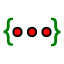# IM1.5.9 Explore function transformations 1: Range

Author:
Lind, Jon
Topic:
Functions

Today, we're going to explore how to change the graph of a function by adding to the function's equation. Below, fill in the columns for the range of the two functions with the given domain. Use the following rules for your functions: When you've found all of the range values for f and g, graph the points on the coordinate plane by selecting both columns (x and f(x)) and creating a list of points1. Look at the individual points you graphed above. How do the outputs from g differ from the outputs from f? 2. What appears to be the effect of subtracting 3 from a function? 3. Make a conjecture: what would happen if you graphed a new function ? Test your conjecture by graphing h(x) above (type |x|+2 in an empty cell) 4. Generalize: How does the graph of f(x) compare to the graph of f(x)+k? (use your vocabulary from geometry!)

## Multiplying the Range

Below, fill in the columns for the range of the two functions with the given domain. Use the following rules for your functions: When you've found all of the range values for f, a, and b, graph the points on the coordinate plane by selecting both columns (x and f(x), for example) and creating a list of points

1. Look at the individual points you graphed above. How do the outputs from a and b differ from the outputs from f? 2. What appears to be the effect of multiplying the outputs of a function? 3. Generalize: How does the graph of f(x) compare to the graph of k·f(x)? (might need some new vocabulary for this one)